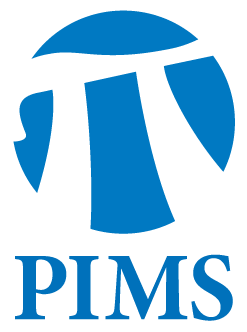## Probability Seminar: Codina Cotar

• Date: 04/18/2012
• Time: 15:00
Lecturer(s):
Codina Cotar
Location:

University of British Columbia

Topic:

Density functional theory and optimal transport with Coulomb cost

Description:

Abstract

In this talk I explain a promising and previously unnoticed link between
electronic structure of molecules and optimal transportation (OT), and I
give some
first results. The `exact' mathematical model for electronic structure,
the many-electron Schroedinger equation, becomes computationally
unfeasible for more than a dozen or so electrons. For larger systems,
the standard model underlying a huge literature in computational
physics/chemistry/materials science is density functional theory (DFT).
In DFT, one only computes the single-particle density instead of the
full many-particle wave function. In order to obtain a closed equation,
one needs a closure assumption which expresses the pair density in terms
of the single-particle density rho.

We show that in the semiclassical Hohenberg-Kohn limit, there holds an
exact closure relation, namely the pair density is the solution to a
optimal transport problem with Coulomb cost. We prove that for the case
with $N=2$ electrons this problem has a unique solution given by an
optimal map; moreover we derive an explicit formula for the optimal map
in the case when $\rho$ is radially symmetric (note: atomic ground state
densities are radially symmetric for many atoms such as He, Li, N, Ne,
Na, Mg, Cu).

In my talk I focus on how to deal with its main mathematical novelties
(cost decreases with distance; cost has a singularity on the diagonal). I
also discus the derivation of the Coulombic OT problem from the
many-electron Schroedinger equation for the case with $N\ge 3$
electrons, and give some results and explicit solutions for the
many-marginals OT problem.

Joint works with Gero Friesecke (TU Munich) and Claudia Klueppelberg (TU Munich).

Other Information:

Location: WMAX 110